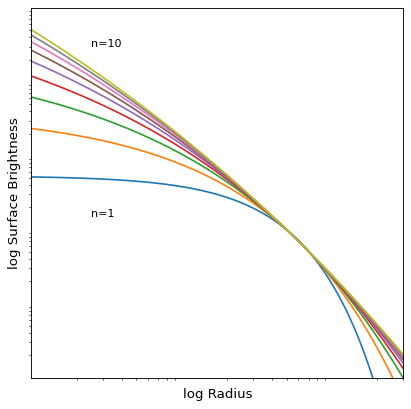# Sersic1D¶

class astropy.modeling.functional_models.Sersic1D(amplitude=1, r_eff=1, n=4, **kwargs)[source]

One dimensional Sersic surface brightness profile.

Parameters
amplitudefloat

Surface brightness at r_eff.

r_efffloat

nfloat

Sersic Index.

Other Parameters
fixeda dict, optional

A dictionary {parameter_name: boolean} of parameters to not be varied during fitting. True means the parameter is held fixed. Alternatively the fixed property of a parameter may be used.

tieddict, optional

A dictionary {parameter_name: callable} of parameters which are linked to some other parameter. The dictionary values are callables providing the linking relationship. Alternatively the tied property of a parameter may be used.

boundsdict, optional

A dictionary {parameter_name: value} of lower and upper bounds of parameters. Keys are parameter names. Values are a list or a tuple of length 2 giving the desired range for the parameter. Alternatively, the min and max properties of a parameter may be used.

eqconslist, optional

A list of functions of length n such that eqcons[j](x0,*args) == 0.0 in a successfully optimized problem.

ineqconslist, optional

A list of functions of length n such that ieqcons[j](x0,*args) >= 0.0 is a successfully optimized problem.

Notes

Model formula:

$I(r)=I_e\exp\left\{-b_n\left[\left(\frac{r}{r_{e}}\right)^{(1/n)}-1\right]\right\}$

The constant $$b_n$$ is defined such that $$r_e$$ contains half the total luminosity, and can be solved for numerically.

$\Gamma(2n) = 2\gamma (b_n,2n)$

References

R3df0f842ba5c-1

http://ned.ipac.caltech.edu/level5/March05/Graham/Graham2.html

Examples

import numpy as np
from astropy.modeling.models import Sersic1D
import matplotlib.pyplot as plt

plt.figure()
plt.subplot(111, xscale='log', yscale='log')
s1 = Sersic1D(amplitude=1, r_eff=5)
r=np.arange(0, 100, .01)

for n in range(1, 10):
s1.n = n
plt.plot(r, s1(r), color=str(float(n) / 15))

plt.axis([1e-1, 30, 1e-2, 1e3])
plt.ylabel('log Surface Brightness')
plt.text(.25, 1.5, 'n=1')
plt.text(.25, 300, 'n=10')
plt.xticks([])
plt.yticks([])
plt.show()


()Attributes Summary

 amplitude input_units This property is used to indicate what units or sets of units the evaluate method expects, and returns a dictionary mapping inputs to units (or None if any units are accepted). n param_names r_eff

Methods Summary

 evaluate(r, amplitude, r_eff, n) One dimensional Sersic profile function.

Attributes Documentation

amplitude
input_units

This property is used to indicate what units or sets of units the evaluate method expects, and returns a dictionary mapping inputs to units (or None if any units are accepted).

Model sub-classes can also use function annotations in evaluate to indicate valid input units, in which case this property should not be overridden since it will return the input units based on the annotations.

n
param_names = ('amplitude', 'r_eff', 'n')
r_eff

Methods Documentation

classmethod evaluate(r, amplitude, r_eff, n)[source]

One dimensional Sersic profile function.